Home

ARTICLE 24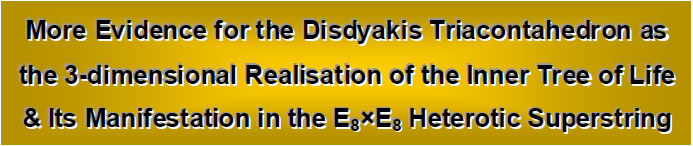by

Stephen M. Phillips

Flat 4, Oakwood House, 117-119 West Hill Road. Bournemouth. Dorset BH2 5PH. England.

Website: http://smphillips.mysite.com

Abstract

 Article 22 presented evidence that the disdyakis triacontahedron represents the 3-dimensional manifestation of the exterior aspect of the 2-dimensional, inner form of the Tree of Life — the geometrical representation of “Adam Kadmon,” or Divine Man. Its 62 vertices define 28 regular and semi-regular solids. Constructed from Pythagorean tetractyses, the template of sacred geometry, they are made up of 3360 hexagonal yods. This number is the number of yods in the seven enfolded, regular polygons forming half of the inner Tree of Life. It is further confirmation that the disdyakis triacontahedron is the 3-dimensional realisation of the inner Tree of Life. Its manifestation in superstring space-time are the 3360 circularly polarised oscillations made during one complete revolution by the ten component closed curves of the E8×E8 heterotic superstring, as described 109 years ago by the Theosophists Annie Besant and C.W. Leadbeater.

1. Introduction
The author has discovered that what is known to Kabbalists as the ‘Tree of Life’ is actually only the outer form of the complete, sacred geometrical system embodying the divine paradigm. It has an inner form, and Articles 22 and 23 showed that the Catalan solid called the ‘disdyakis triacontahedron’ is its polyhedral manifestation. Through the equivalence between the (6n+1) yods of an n-sided, regular polygon with its sectors turned into tetractyses and the (6n+1) Sephirothic emanations up to Chesed of the highest tree in n overlapping Trees of Life, this inner form can be shown1 to encode the replication of its outer form to map all levels of reality, both physical space-time and

1

Table 1. Gematria number values of the 10 Sephiroth in the four Worlds.

 SEPHIRAH GODNAME ARCHANGEL ORDER OF ANGELS MUNDANE CHAKRA 1 Kether (Crown)                  620 EHYEH (I am)                  21 Metatron (Angel of the Presence)                  314 Chaioth ha Qadesh (Holy Living Creatures)                  833 Rashith ha Gilgalim First Swirlings. (Primum Mobile)                  636 2 Chokmah (Wisdom)                  73 YAHWEH, YAH (The Lord)                  26, 15 Raziel (Herald of the Deity)                  248 Auphanim (Wheels)                  187 Masloth (The Sphere of the Zodiac)                  140 3 Binah (Understanding)                  67 ELOHIM (God in multiplicity)                  50 Tzaphkiel (Contemplation of God)                  311 Aralim (Thrones)                  282 Shabathai Rest. (Saturn)                  317 Daath (Knowledge)                  474 4 Chesed (Mercy)                  72 EL (God)                  31 Tzadkiel (Benevolence of God)                  62 Chasmalim (Shining Ones)                  428 Tzadekh Righteousness. (Jupiter)                  194 5 Geburah (Severity)                  216 ELOHA (The Almighty)                  36 Samael (Severity of God)                  131 Seraphim (Fiery Serpents)                  630 Madim Vehement Strength. (Mars)                  95 6 Tiphareth (Beauty)                  1081 YAHWEH ELOHIM (God the Creator)                  76 Michael (Like unto God)                  101 Malachim (Kings)                  140 Shemesh The Solar Light. (Sun)                  640 7 Netzach (Victory)                  148 YAHWEH SABAOTH (Lord of Hosts)                  129 Haniel (Grace of God)                  97 Tarshishim or Elohim                  1260 Nogah Glittering Splendour. (Venus)                  64 8 Hod (Glory)                  15 ELOHIM SABAOTH (God of Hosts)                  153 Raphael (Divine Physician)                  311 Beni Elohim (Sons of God)                  112 Kokab The Stellar Light. (Mercury)                  48 9 Yesod (Foundation)                  80 SHADDAI EL CHAI (Almighty Living God)                  49, 363 Gabriel (Strong Man of God)                  246 Cherubim (The Strong)                  272 Levanah The Lunar Flame. (Moon)                  87 10 Malkuth (Kingdom)                  496 ADONAI MELEKH (The Lord and King)                  65, 155 Sandalphon (Manifest Messiah)                  280 Ashim (Souls of Fire)                  351 Cholem Yesodoth The Breaker of the Foundations. The Elements. (Earth)                  168

 The Sephiroth exist in the four Worlds of Atziluth, Beriah, Yetzirah and Assiyah. Corresponding to them are the Godnames, Archangels, Order of Angels and Mundane Chakras (their physical manifestation). This table gives their number values obtained by the ancient practice of gematria, wherein a number is assigned to each letter of the alphabet, thereby giving a number value to a word that is the sum of the numbers of its letters.

(All numbers from this table that are referred to in the article are written in boldface).

2

superphysical realms. The seven enfolded, regular polygons constituting one half of the inner Tree of Life map what are known in Theosophy as the 49 subplanes of the seven planes of consciousness: physical, astral, mental, buddhic, atmic, anupadaka (or Monadic) and adi (or Divine), each subplane being represented by its own Tree of Life. The 120 yods on theboundaries of the seven polygons signify the 120 faces of the disdyakis triacontahedron and the 144 yods inside them denote the 144 faces of another polyhedron (Fig. 1). This ‘144 Polyhedron’ was identified in Article 23 as generated from the disdyakis dodecahedron (one of the 13 Catalan solids), which has 48 faces, 26 vertices & 72 edges. This is the only semi-regular polyhedron that has 48 faces, which means that it, alone, can generate a polyhedron with 144 faces when tetrahedra are stuck on its faces.

2. Polyhedral content of the disdyakis triacontahedron
The 62 vertices of the disdyakis triacontahedron define the vertices of 28 regular and semi-regular solids: ten tetrahedra, five cubes, five octahedra, one icosahedron, one dodecahedron, five rhombic dodecahedra and one rhombic triacontahedron. A rhombic dodecahedron (Fig. 2) is an Archimedean solid with 14 vertices, 24 edges and 12 rhombic faces. Constructed from four tetractyses, each of the 12 rhombic faces has 12 internal hexagonal yods, whilst two hexagonal yods lie on each of its 24 edges. The number of hexagonal yods in the rhombic dodecahedron is therefore 2×24 + 12×12 = 192 (see Article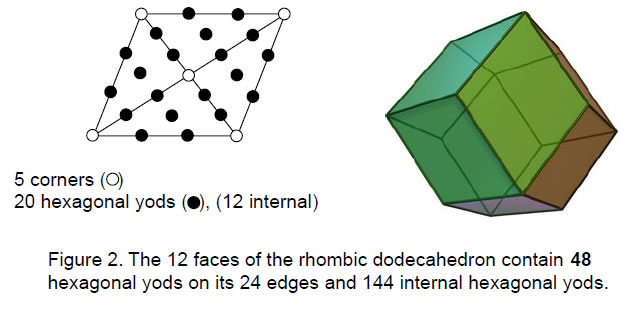3

18 for the significance of this parameter of holistic systems vis-à-vis the I Ching table and the Bode numbers of the planets). When the five, separate rhombic dodecahedra group, their (5×14=70) separate vertices become the 62 vertices and raised centres of the 30 Golden Rhombic faces of the disdyakis triacontahedron, their 30 unshared vertices coinciding with the centres of these faces and their 32 shared vertices coinciding with their vertices (Fig. 3). Each rhombic dodecahedron has six unshared vertices and eight shared vertices that are vertices of these faces. Each face vertex coincides with two vertices of different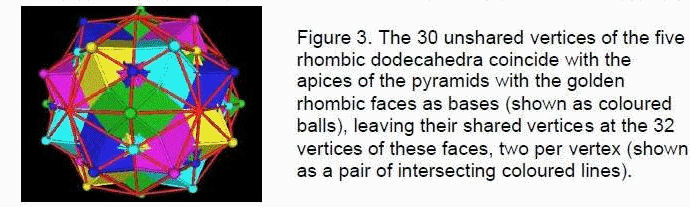dodecahedra:

 A1B1 A2C1 A3D1 A4E1 A5B2 A6C2 A7D2 A8E2 B3C3 B4D3 B5E3 B6C4 B7D4 B8E4 C5D5 C6E5 C7D6 C8E6 D7E7 D8E8

where the letters label the five dodecahedra and the numbers indicate their vertices.

Let us now construct the 28 solids from tetractyses — the template of sacred geometry — and then work out their populations of hexagonal yods. The significance of the latter is that the seven hexagonal yods of the tetractys symbolise the seven Sephiroth of Construction, the formative degrees of freedom expressing the 'objective' aspects of God). The hexagonal yod populations of the 28 polyhedra in the disdyakis triacontahedron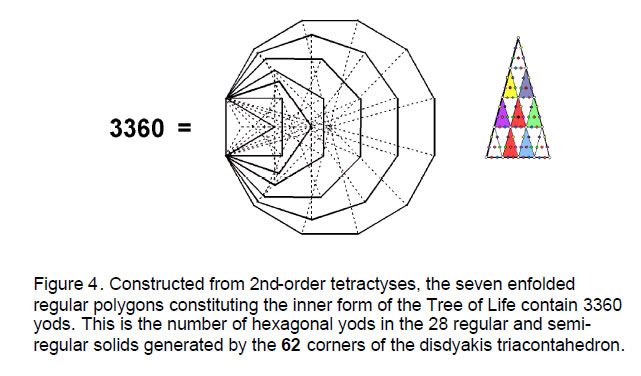4

are:2

 tetrahedron: 10×48 = 480 cube: 5×96 = 480 octahedron: 5×96 = 480 icosahedron: 1×240 = 240 dodecahedron: 1×240 = 240 rhombic dodecahedron: 5×192 = 960 rhombic triacontahedron: 1×480 = 480 Total = 3360

This result is truly astounding for two complementary reasons:

1. Divine Unity symbolised by the Pythagorean Monad, or mathematical point ("0th-order tetractys"), differentiates, firstly, into the familiar tetractys ("1st-order tetractys") with 10 yods (three corners, seven hexagonal yods), secondly, into the “2nd-order tetractys” with 85 yods (15 corners, 70 hexagonal yods), and so on: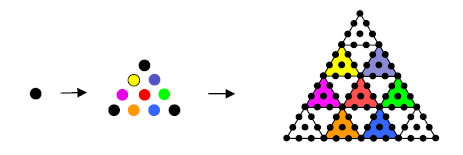3360 is the number of yods in the seven enfolded, regular polygons constituting the inner form of the Tree of Life when their 47 sectors are each turned into the 2nd-order tetractys (Fig. 4).3 We now see that the seven types of solids terminating in the disdyakis triacontahedron contain 1680 hexagonal yods (as shown on page 14 of Article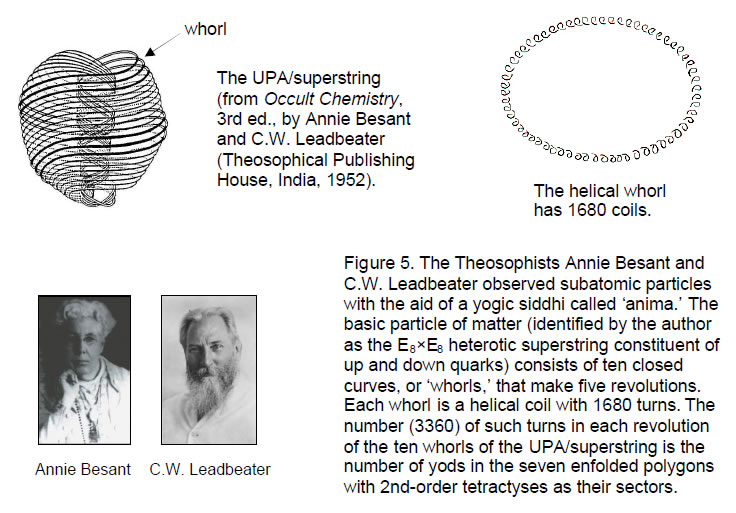5

224 ), whilst the actual numbers for the seven types of solids in the disdyakis triacontahedron total 3360 hexagonal yods, where 3360 = 2×1680. This is the total number of yods making up the polygonal, inner form of the Tree of Life constructed from the template of the 2nd-order tetractys. The number 3360 expresses a holistic structure both in the 2-dimensional space of the polygons and in the 3-dimensional space of the disdyakis triacontahedron. This marvellous, beautiful property of the polyhedron clearly demonstrating its Tree of Life basis!

2. 3360 is the number of circularly polarised standing wave oscillations made during each of the five revolutions of the ten closed curves making up the E8×E8 heterotic superstring, as described by Annie Besant and C.W. Leadbeater in 1908, when they used anima to magnify subatomic particles (Fig. 5), whilst 1680 is the number of such oscillations in each curve. What this means is that, as the completion of the seven-fold sequence of regular and semi-regular polyhedra, the disdyakis triacontahedron has to be made up of the same number of formative degrees of freedom (hexagonal yods) as there are yods needed to represent its 2-dimensional counterpart, namely, the seven enfolded, regular polygons. Far from being a coincidence, the presence of the same number in two superficially different contexts reveals in an unambiguous way the beautiful, mathematical design of a transcendental, creative Intelligence. To discover this mathematical harmony, we need to understand 'sacred geometry' — not the distorted version found in many books, which lack understanding of the fundamental principles — but the only geometry worthy of being called 'sacred,’ namely, that of the Tree of Life.

Each of the 42 sides of the seven enfolded polygons has 11 yods between their ends, which number 36. The number of yods forming the boundaries of the polygons = 11×42 + 36 = 498. In other words, 496 yods form the sides of the polygons between the two endpoints of the root edge that generates them. This is the number value of the Hebrew word ‘Malkuth’ signifying the last Sephirah of the Tree of Life. It is yet another confirmation that the disdyakis triacontahedron is the outer (or Malkuth) aspect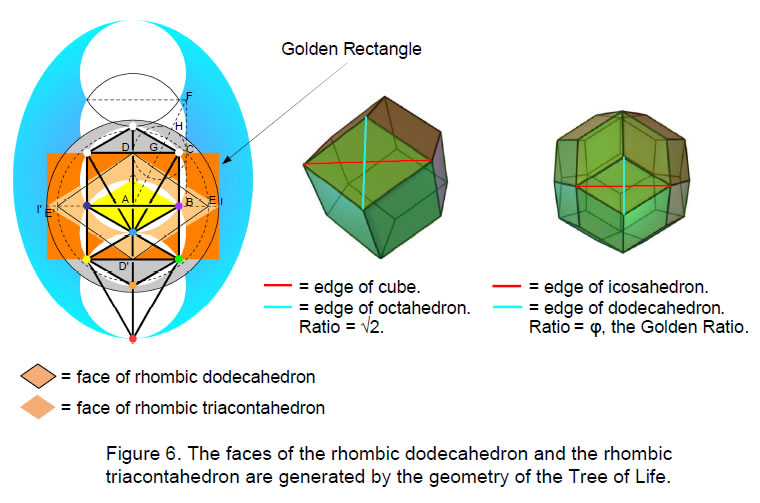6

of the inner Tree of Life. As discovered by physicists Michael Green and John Schwarz5 in 1984, 496 is the dimension of the non-abelian gauge symmetry group defining superstring interactions that are free of quantum anomalies. We therefore encounter the following amazing property of the polygonal form of the inner Tree of Life blueprint: it encodes not only the oscillatory pattern of the E8×E8 heterotic superstring but also the number of gauge bosons that transmit its unified force — the first as its yod population and the second as the number of yods forming its boundary between the endpoints of its generative root edge.

3. The rhombic dodecahedron and rhombic triacontahedron
The rhombic dodecahedron has 12 rhombic faces (Fig. 6). The longer diagonal of each face (shown as a red line in Fig. 6) is the edge of a cube and the shorter diagonal (shown as a blue line) is the edge of an octahedron. The ratio of the lengths of the longer and shorter diagonals is √2 = 1.414... . These Platonic solids are dual to each other. The two other Platonic solids that are dual to one another — the icosahedron and the dodecahedron — share an analogous property in that their edges are, respectively, the longer and shorter diagonals of the faces of the rhombic triacontahedron. Their ratio is the Golden Ratio φ = 1.618… .These rhomboids are generated in a simple way by the geometry of the Tree of Life. Fig. 6 indicates how the ten Sephiroth are the centres or points of intersection of a column of white circles. Let us take their radii as one unit. The central Pillar of Equilibrium intersects the path joining Chesed and Geburah at a point A that is one unit away from the vertical right-hand tangent BC to these circles. ABCD is a square with sides of length 1. Therefore, its diagonal AC = √(12 + 12) = √2. With A as centre, draw a circle passing through C of radius √2 (shown as a dashed line in Fig. 6). It intersects the line drawn along AB at E. E' is the corresponding point on the other side of the central pillar. EE' = 2√2. The central pillar intersects the path joining Netzach and Geburah at D'. DD' = 2. Therefore, EE'/DD' = 2√2/2 = √2. We find that the rhombus DED'E' has the same shape as the rhombic face of the rhombic dodecahedron.

Extend the tangent at B to the point F, where BC = CF = 1. Then, BF = 2 and AF = √(22 + 12) = √5. The line AF intersects CD at G, where AG = GF = √5/2. With G as centre, draw a circle of radius ½. It intersects AF at H, where AH = √5/2 + ½ = (√5+1)/2 = φ, the Golden Ratio. With A as centre, draw a circle of radius AH. It intersects the extension of AB at I, where AI = φ. I' is its counterpart on the other side of the central pillar. II' = 2φ. Therefore, II'/DD' = 2φ/2 = φ. The rhombus DI D'I' has the same shape as the rhombic face of the rhombic triacontahedron. What manifests finally as the fruit of the Tree of Life, namely, the disdyakis triacontahedron with golden rhombic faces, was within it as their seed shape from the very beginning!

References
1 Phillips, Stephen M. The Mathematical Connection between Religion and Science (Antony Rowe Publishing, England, 2009).

2 The hexagonal yod populations of these solids are taken from Article 22: “The disdyakis triacontahedron as the 3-dimensional counterpart of the Inner Tree of Life,” Stephen M. Phillips, (WEB, PDF), p. 6.

3 Proof: The 2nd-order tetractys has 85 yods, of which 13 yods line each of its sides. When each of the n triangular sectors of an n-sided, regular polygon are turned into a 2nd-order tetractys, there are (85–13=72) independent yods per sector of the polygon. Its yod population = 72n + 1, where “1” denotes the yod at the centre of the polygon. The polygonal form of the inner Tree of Life consists of a triangle, square, pentagon, hexagon, octagon, decagon and dodecagon. They are enfolded in one another and share the same base, or what the author has called the “root edge,” as they should be thought of as

7

growing out of this fundamental line joining Daath and Tiphareth in the Tree of Life. When the seven separate polygons are superposed on one another in their enfolded state, corresponding members of the set of 13 yods forming what becomes their shared side coincide and therefore must not be counted separately in a calculation of their yod population. Below are listed the yod populations of each polygon and (except for the triangle) their numbers of yods outside the root edge:

 Polygon n Number of yods = 72n + 1 Number of yods outside root edge triangle 3 217 217 square 4 289 289 – 13 =   276 pentagon 5 361 361 – 13 =   348 hexagon 6 433 433 – 13 =   420 octagon 8 577 577 – 13 =   564 decagon 10 721 721 – 13 =   708 dodecagon 12 865 865 – 13 =   852 Total = 3385

Inspection of Fig. 4 reveals that the tip of the triangle viewed with the root edge as its base is also the centre of the hexagon (the triangle is simply a triangular sector of the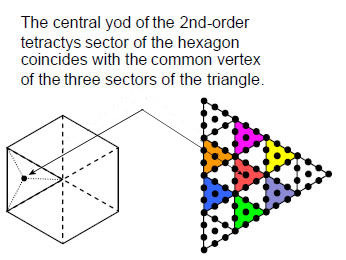hexagon). Similarly, the tip of the pentagon is the centre of the decagon. With 2nd-order tetractyses as their sectors, the centroid of the triangle where corners of its three 2nd-order tetractyses meet is also the central yod of the tetractys at the centre of the 2nd-order tetractys constituting a sector of the hexagon (see diagram). The 11 yods between corners on each of the two sides of the triangle outside its shared base coincide with yods on the sides of this sector of the hexagon. There are (1 + 1 + 1 + 2×11 = 25) yods in the total population calculated above that coincide with yods belonging to other polygons (these are the only yods occupying the same positions). In determining the yod population when the separate polygons are superposed, these yods must be subtracted in order to avoid double-counting Therefore, the yod population of the seven enfolded polygons constructed from 2nd-order tetractyses = 3385 – 25 = 3360.

4 Ref. 2, p. 14.

5 Green, M.B. & Schwarz, J.H. “Anomaly cancellations in supersymmetric d = 10 gauge theory and superstring theory.” Physics Letters, B149, 117.

8

Home